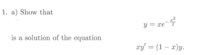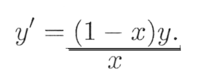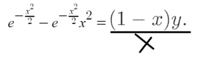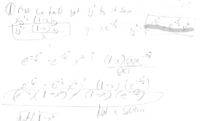# solutions to derivatives, what mistakes am I making?

#### mlw19mlw91

##### New member
guys here's my problemI start by dividing everything by X to get y' by itself

I get(is this the problem?)

then I take the derivative of the Y that he gave us, wich was the y+xe^((-x^2)/2)
and I get the following:
e^{-(x^2}/{2}}-e^{-{x^2}/{2}}x^2

now I put this on the left hand side of the equation, and I get something like this:then then I plug in the y that he originally gave us, I can not end up with a true statement.

Here's my work on paper, and please forgive me for the messiness:#### ksdhart2

##### Full Member
Well, I agree with your derivative:

$$\displaystyle y = xe^{-\frac{x^2}{2}} \implies \frac{dy}{dx} = -e^{-\frac{x^2}{2}} \cdot (x^2 - 1)$$

So let's plug that into the solution and see if checks:

$$\displaystyle xy^{\prime} \stackrel{?}{=} (1-x)y$$

$$\displaystyle x \cdot -e^{-\frac{x^2}{2}} \cdot (x^2 - 1) \stackrel{?}{=} (1-x)y$$

$$\displaystyle -e^{-\frac{x^2}{2}} \cdot x(x^2 - 1) \stackrel{?}{=} (1-x) \cdot xe^{-\frac{x^2}{2}}$$

$$\displaystyle -e^{-\frac{x^2}{2}} \cdot x(x - 1)(x + 1) \stackrel{?}{=} (1-x) \cdot xe^{-\frac{x^2}{2}}$$

Assuming $$\displaystyle x \ne 0$$ and $$\displaystyle x \ne 1$$, we can divide both sides by $$\displaystyle x$$ and $$\displaystyle (x- 1)$$:

$$\displaystyle -e^{-\frac{x^2}{2}} \cdot (x + 1) \stackrel{?}{=} -e^{-\frac{x^2}{2}}$$

Oh, that's not good. This means it's only true for some specific values of $$\displaystyle x$$. Even worse, it's specifically only true for exactly the two values we assumed $$\displaystyle x$$ wasn't equal to! WolframAlpha confirms this result.

About the only thing I can possibly conclude from all of this is that the problem is impossible. We can't show that the given $$\displaystyle y$$ is a solution to the given equation, because it's not. Perhaps there's a typo in the exercise somewhere?

•topsquark

#### MarkFL

##### Super Moderator
Staff member
Hello, and welcome to FMH!The way I would work this problem, is to take the given:

$$\displaystyle y=xe^{-\frac{x^2}{2}}$$

And then compute:

$$\displaystyle y'=e^{-\frac{x^2}{2}}-x^2e^{-\frac{x^2}{2}}=(1-x^2)e^{-\frac{x^2}{2}}$$

Next, substitute for $$y$$ and $$y'$$ into the given ODE:

$$\displaystyle x\left((1-x^2)e^{-\frac{x^2}{2}}\right)=(1-x)\left(xe^{-\frac{x^2}{2}}\right)$$

Divide through by $$x(1-x)e^{-\frac{x^2}{2}}$$:

$$\displaystyle x=0$$

Now, we normally expect to obtain an identity when we do this. If we write the ODE as:

$$\displaystyle y'+\frac{x-1}{x}y=0$$

Note: we are losing the trivial solution associated with $$x=0\implies y=0$$.

Compute the integrating factor:

$$\displaystyle \mu(x)=\exp\left(\int\frac{x-1}{x}\,dx\right)=\frac{e^x}{x}$$

then the ODE becomes:

$$\displaystyle \frac{e^x}{x}y'+\frac{(x-1)e^x}{x^2}y=0$$

$$\displaystyle \frac{d}{dx}\left(\frac{e^x}{x}y\right)=0$$

Integrate:

$$\displaystyle \frac{e^x}{x}y=c_1$$

Hence:

$$\displaystyle y(x)=c_1xe^{-x}$$

Based on this, I wonder if the author of the problem actually intended for you to verify that:

$$\displaystyle y=xe^{-x}$$

is a solution to the given ODE.

•topsquark

#### mlw19mlw91

##### New member
ksdhart2, thank you for confirming my maths! your work seems to confirm my suspicion

MarkFL thank you for your help & answer. We have not learned integration yet, I am not sure, your solution is a little above my head. I think for now I'm going to assume this problem can not be solved with the maths that I currently know

#### MarkFL

##### Super Moderator
Staff member
I really just wanted to find the general solution to find what might be what the author of the problem actually wanted. Or, it could be the author wanted you to discover the given solution doesn't work.

#### Jomo

##### Elite Member
guys here's my problem
View attachment 11389

I start by dividing everything by X to get y' by itself

I get
View attachment 11390
(is this the problem?)

then I take the derivative of the Y that he gave us, wich was the y+xe^((-x^2)/2)
and I get the following:
e^{-(x^2}/{2}}-e^{-{x^2}/{2}}x^2

now I put this on the left hand side of the equation, and I get something like this:

View attachment 11393

then then I plug in the y that he originally gave us, I can not end up with a true statement.

Here's my work on paper, and please forgive me for the messiness:
View attachment 11394
First compute y', then multiply this by x. Hold onto that result.
2nd multiply (1-x) by xex^2/2
These two results should be equal. You might have to change the way the sides look to get them to look identical.